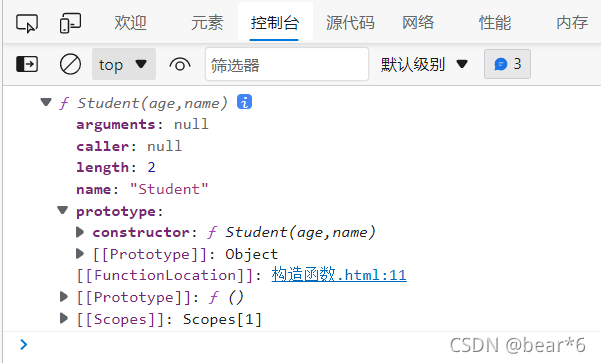﻿ JavaScript构造函数与原型之间的联系_JavaScript_脚本之家
JavaScript# JavaScript构造函数与原型之间的联系

## 一、构造函数和原型

### 1、构造函数

• 构造函数用于创建某一类对象，其首字母要大写。
• 构造函数要和`new `一起使用才有意义。

new 在执行时会做四件事情：

• 在内存中创建一个新的空对象。
• 让 this 指向这个新的对象。
• 执行构造函数里面的代码，给这个新对象添加属性和方法。
• 返回这个新对象（所以构造函数里面不需要 `return `）。

`JavaScript `的构造函数中可以添加一些成员，可以在构造函数本身上添加，也可以在构造函数内部的 this 上添加。通过这两种方式添加的成员，就分别称为静态成员和实例成员。

``` function A(uname,age){
this.uname = uname;
this.age  = age;
this.say = function() {
console.log(this.uname+'你好');
}
}
var wh = new A('王欢',18);
var xl = new A('小熊',18);

```

```A.sex='女';

```

### 2、构造函数的问题

```function Student(age,name){
this.age = age;
this.name = name;
this.score = function(){
console.log('孩子们成绩都很好！');
}
}
console.dir(Student);
var xl = new Student(18,'小熊');
var wh = new Student(17,'王欢');
xl.score();
wh.score();

```

``` console.log(xl.score === wh.score);

```### 3、构造函数原型 prototype

```function Student(age,name){
this.age = age;
this.name = name;
this.score = function(){
console.log('孩子们成绩都很好！');
}
}
console.dir(Student);

``````function Student(age,name){
this.age = age;
this.name = name;
}
Student.prototype.score = function(){
console.log('孩子们成绩都很好！');
}
console.dir(Student);
var xl = new Student(18,'小熊');
var wh = new Student(17,'王欢');
xl.score();
wh.score();
console.log(xl.score === wh.score);

```### 4、对象原型 __proto__

```function Student(age,name){
this.age = age;
this.name = name;

}
Student.prototype.score = function(){
console.log('孩子们成绩都很好！');
}
// console.dir(Student);
var xl = new Student(18,'小熊');
var wh = new Student(17,'王欢');
console.log(xl);

```

```console.log(xl);//对象身上系统自己添加一个__proto__属性指向构造函数的原型对象

`````` console.log(xl.__proto__ === Student.prototype);

```

```xl.score();

````__proto__`对象原型的意义就在于为对象的查找机制提供一个方向，或者说一条路线，但是它是一个非标准属性，因此实际开发中，不可以使用这个属性，它只是内部指向原型对象 `prototype`

### 5、constructor 构造函数

```console.log(Student.prototype);
console.log(xl.__proto__);

```可知：对象原型（ __proto__）和构造函数（prototype）原型对象里面都有一个属性 constructor 属性 ，`constructor`我们称为构造函数，因为它指回构造函数本身。

``` console.log(Student.prototype.constructor);
console.log(xl.__proto__.constructor);

````constructor `主要用于记录该对象引用于哪个构造函数，它可以让原型对象重新指向原来的构造函数。

一般情况下，对象的方法都在构造函数的原型对象中设置。当给构造函数添加多个方法时，可以采用对象的方式，

``` Student.prototype = {
score: function(){
console.log('孩子们成绩都很好！')},
study: function(){
console.log('好好学习！');
}

```发现原型对象的指向发生变化，这是因为给原型对象采取对象形式赋值，这样就会覆盖构造函数原型对象原来的内容，这样修改后的原型对象`constructor `就不再指向当前构造函数了。

```Student.prototype = {
constructor:Student,
score: function(){
console.log('孩子们成绩都很好！')},
study: function(){
console.log('好好学习！');
}

```### 6、构造函数、实例、原型对象三者之间的关系### 7、JavaScript 的成员查找机制(规则)

• 当访问一个对象的属性（包括方法）时，首先查找这个对象自身有没有该属性。
• 如果没有就查找它的原型（也就是 __proto__指向的 `prototype`原型对象）。
• 如果还没有就查找原型对象的原型（Object的原型对象）。

__proto__对象原型的意义就在于为对象成员查找机制提供一个方向，或者说一条路线。

### 8、 扩展内置对象

``` console.log(Array.prototype);

`````` Array.prototype.sum = function(){
var sum = 0;
for(var i=0;i<this.length;i++){
sum += this[i];
}
return sum;
}

```

```console.log(Array.prototype);

``````var arr = [1,2,3];
console.log(arr.sum());

```## 二、类的本质

• `class`本质还是`function`.
• 类的所有方法都定义在类的`prototype`属性上
• 类创建的实例,里面也有__proto__ 指向类的prototype原型对象
• ES6的类它的绝大部分功能，ES5都可以做到，新的`class`写法只是让对象原型的写法更加清晰、更像面向对象编程的语法而已。